# Python Insertion Sort

Here you’ll learn about Python insertion sort algorithm.

In terms of performance Insertion sort is not the best sorting algorithm. But it is little bit more efficient then the Selection sort and Bubble sort.

To understand the Insertion Sort algorithm easily, we’ll start with an example.

## Python Insertion Sort

### Example

Let’s say we have an array [14,5,18,6,21].

Assume that we have two parts in this array first one is sorted and another one is unsorted.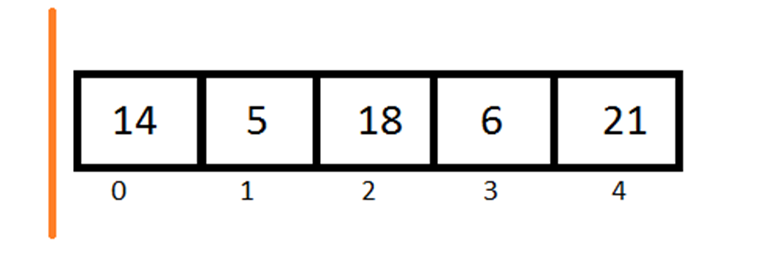The  sorted part of array is in the left of orange line and unsorted part is in the right side.

Now all we have to do is pick each element one by one from the unsorted part and add it into the sorted array at its appropriate place.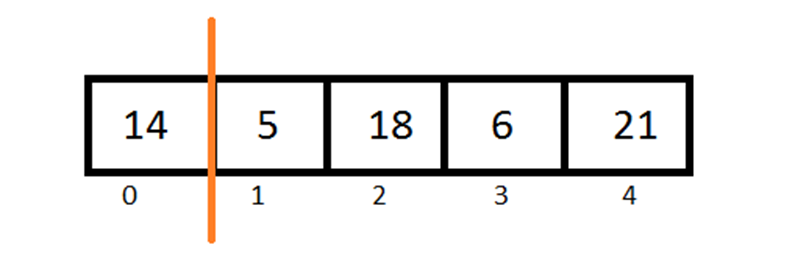14 is the only element in our sorted part so it is already sorted.

Now pick the first element from the unsorted part and add it to the sorted part.As 5 is less than 14 so 14 have to right shift by one place.

Again pick first element from unsorted part and add it into in sorted part.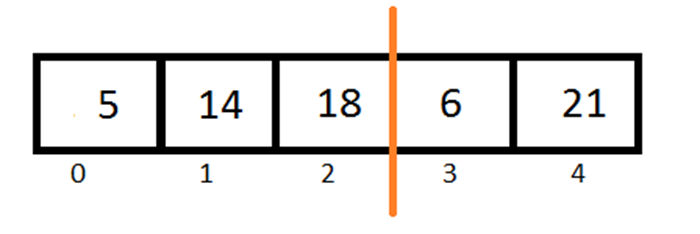18 is greater than 14 and there is no need to shifting.

Repeating same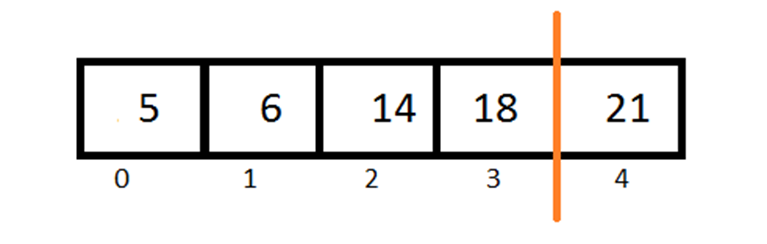6 is greater than 5 and less than 14.

So we have to right shift 14 and 18 by one place.

At last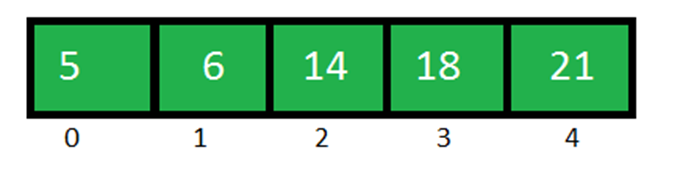21 is greater than 18 doesn’t need to shift. Done.

### Algorithm

We have an array ‘Arr[]’ with n elements

Step 1:  set i = 1

Step 2: set value = Arr[i]

Set position = i

Step3 :  if position > 0 and Arr[position-1]>value

Arr[position] = Arr[position-1]

Position = position -1

[Repeat this step until condition become false]

Step 4: Arr[position] = value

Step 5: goto step 2 for (n-1) times.

### Program for Insertion Sort in Python

Output

5 6 14 18 21

Comment below if you have any queries related to above python insertion sort algorithm.